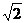## Abstract Database

#### 3D MODELING OF DYNAMIC RUPTURE PROPAGATION BASED ON A BOUNDARY INTEGRAL EQUATION METHOD FOR EARTHQUAKES IN CHINA

MEE10501
Zhang Lifen
We developed a code for dynamic rupture propagation on arbitrarily shaped faults based on the stress Green’s function corresponding to a triangular mesh fault element. A boundary integral equation method is adopted in this study. Starting from representative theorem, after regularization and descretization of a basic elastodynamic equation relating stress with slip, a more applicable formulation used in dynamic rupture simulation is constructed. For a boundary condition, we utilize a simple but typical slip weakening friction law. In order to avoid numerical oscillation, according to Courant-Friedrichs-Lewy (CFL) condition, time intervals used in the simulation is given as Δt≤l/6CL (l is the element size, and CL denotes P wave velocity). And then we applied the code to a planar fault and a curved fault.